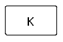## Constant Key Assignment Help

Assignment Help: >> Calculator Operations - Constant Key

Constant KeyCertain calculations frequent contain repetitive operations and numbers. The K, constant, is a time-saving function which allows a single key stroke to perform a single operation and number on the displayed number.

For example, if 20 numbers are to be multiplied by -17.35, the K key can be used. Enter  -17.35,  then  press  the  times  key,  then  the  K  key;  this  "teaches"  the calculator the needed operation. From this point on while entering a number and pressing  the  K  key,  the  calculator  will  automatically  multiply  the  displayed number through -17.35, saving you six key strokes.